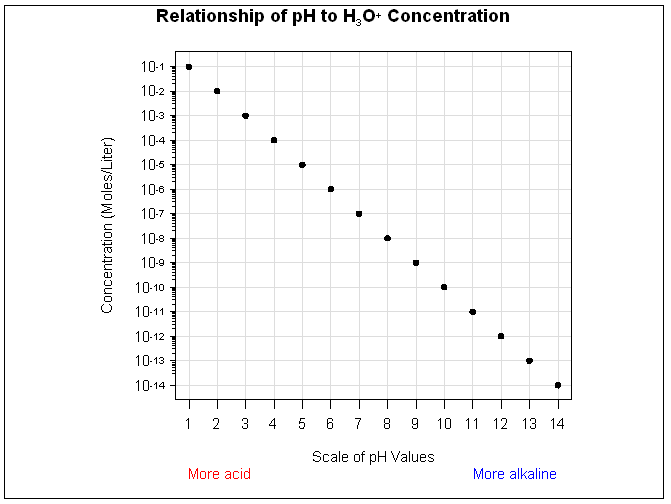SAS/GRAPH Statements

Example 2. Specifying Logarithmic Axes

Features:

AXIS statement options:

 LABEL= LENGTH= LOGBASE= LOGSTYLE= MAJOR= MINOR= VALUE=

TITLE statement option:

 MOVE=

GOPTIONS statement options:

 GUNITThis example illustrates the AXIS statement options LOGBASE= and LOGSTYLE=. The horizontal axis represents pH level. The vertical axis, which represents the concentration of the hydroxide ion expressed as moles per liter, is scaled logarithmically. In addition, this example shows how the TICK= parameter of the VALUE= option modifies individual tick marks.

The example uses the MOVE= option in a TITLE statement to position the title's subscript and superscript text.

Set the graphics environment. The GUNIT option specifies the default unit of measure to use with height specifications.

goptions reset=all  gunit=pct;

Create the data set. The CONCENTR option contains the pH values and the concentration amount.

data concentr;
input ph conc;
datalines;
1  1E-1
2  1E-2
3  1E-3
4  1E-4
5  1E-5
6  1E-6
7  1E-7
8  1E-8
9  1E-9
10 1E-10
11 1E-11
12 1E-12
13 1E-13
14 1E-14
;

run;

Define title and footnote. The MOVE= option positions subscript 3 and superscript +. Each new position is relative to the last position specified by the MOVE= option.

title1 h=3.7 "Relationship of pH to H"
move=(-0,-.75) h=2 "3"
move=(+0,+.75) h=2 "O"
move=(+0,+.75) h=2 "+"
move=(-0,-.75) h=2  " Concentration";

Define symbol characteristics.

symbol value=dot color=black height=2;

Define characteristics for horizontal axis. The LABEL= option uses the JUSTIFY= suboption to create a descriptive two-line label that replaces the variable name PH. MINOR=NONE removes all minor tick marks. The LENGTH= option controls the length of the horizontal axis. The OFFSET= option specifies the distance from the first and last major tick marks to the ends of the axis line.

axis1 label=(h=3 "Scale of pH Values"
justify=left color=red h=2 "More acid"
justify=right color=blue "More alkaline")
minor=none
length=60
offset=(2,2);

Define characteristics for vertical axis. LOGBASE=10 scales the vertical axis logarithmically, using a base of 10. Each major tick mark represents a power of 10. LOGSTYLE=EXPAND displays minor tick marks in logarithmic progression. The LABEL= option uses the ANGLE= suboption to place the label parallel to the vertical axis. The VALUE= option displays the major tick mark values as 10 plus an exponent. The HEIGHT= suboption for each TICK= specification affects only the text following it.

axis2 logbase=10
logstyle=expand
label=(angle=90 h=2 color=black
"Concentration (Moles/Liter)" )
value=(tick=1 "10" height=1.2 "-14"
tick=2 "10" height=1.2 "-13"
tick=2 "10" height=1.2 "-13"
tick=3 "10" height=1.2 "-12"
tick=4 "10" height=1.2 "-11"
tick=5 "10" height=1.2 "-10"
tick=6 "10" height=1.2 "-9"
tick=7 "10" height=1.2 "-8"
tick=8 "10" height=1.2 "-7"
tick=9 "10" height=1.2 "-6"
tick=10 "10" height=1.2 "-5"
tick=11 "10" height=1.2 "-4"
tick=12 "10" height=1.2 "-3"
tick=13 "10" height=1.2 "-2"
tick=14 "10" height=1.2 "-1")
offset=(3,3);

Generate the plot and assign AXIS definitions. AXIS1 modifies the horizontal axis and AXIS2 modifies the vertical axis. The AUTOHREF and AUTOVREF options draw reference lines at all major tick marks on both axes. The CHREF and CVREF options specify the color for these reference lines.

proc gplot data= concentr;
plot conc*ph / haxis=axis1
vaxis=axis2
autohref chref=graydd
autovref cvref=graydd;
run;
quit;Previous Page | Next Page | Top of Page